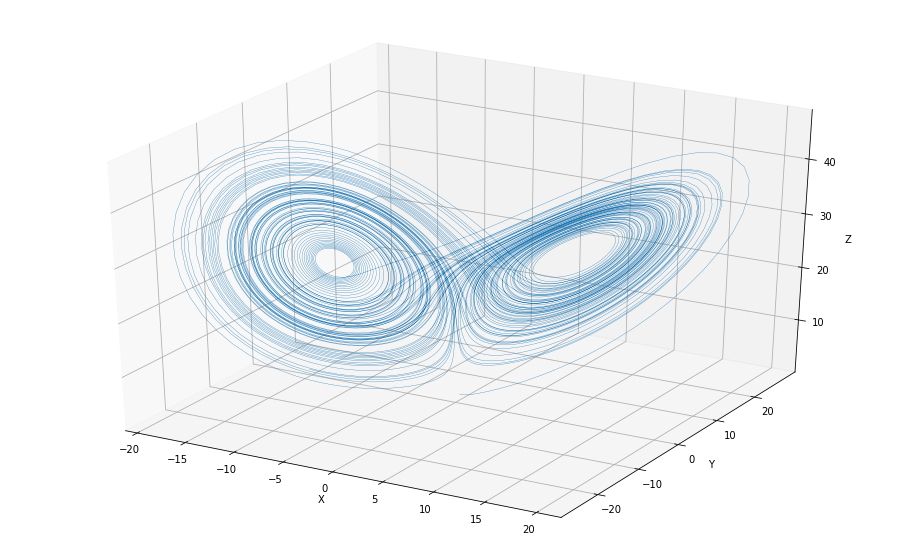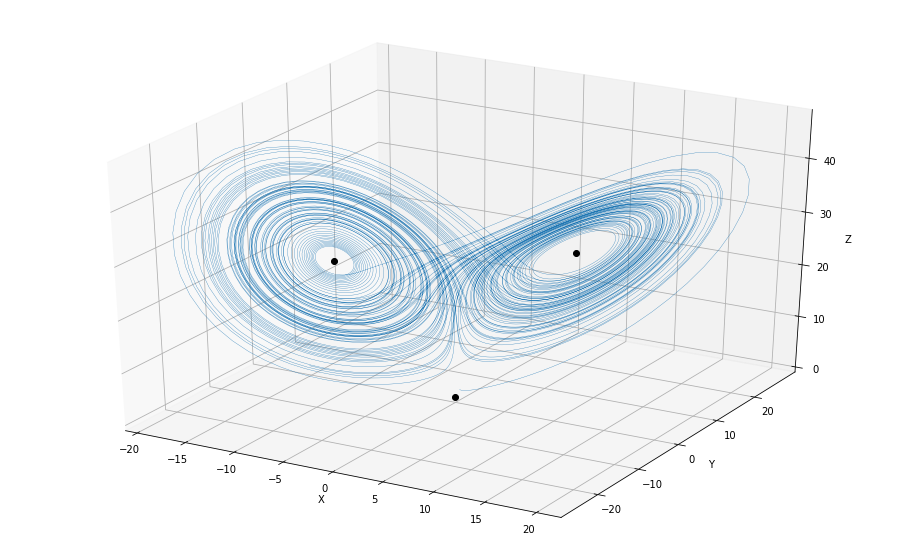# The Lorenz equations¶

Here's a quick exploration of the Lorenz equations with Python. Of course, we'll use some libraries:

In :
import matplotlib.pyplot as plt
from mpl_toolkits.mplot3d import Axes3D
import numpy as np
from scipy.integrate import odeint
from scipy.optimize import root
from scipy.linalg import eig


Mathematically, the Lorenz equations are:

\begin{align} x' &= \sigma(y-x) \\ y' &= x(\rho-z)-y \\ z' &= xy-\beta z. \end{align}

The are many interesting values for the parameters; we'll take them to be

$$\sigma = 10, \: \rho = 28, \text{ and } \: \beta=8/3.$$

Here's a plot of a solution starting near the origin:

In :
def lorenz(xyz, t=0, s=10, r=28, b=8/3):
x,y,z = xyz
xp = s*(y - x)
yp = r*x - y - x*z
zp = x*y - b*z
return xp, yp, zp
ts = np.linspace(0,100,10000)
sol = odeint(lorenz, [0,1,1.05], ts)
xs,ys,zs = sol.T

fig = plt.figure(figsize=(16,10))
ax = fig.gca(projection='3d')
ax.plot(xs, ys, zs, linewidth=0.3)
ax.set_xlabel("X")
ax.set_ylabel("Y")
ax.set_zlabel("Z")
plt.show()We can plot this together with the equilibria:

In :
fig = plt.figure(figsize=(16,10))
ax = fig.gca(projection='3d')

r0 = root(lorenz, [0,0,0]).x
r1 = root(lorenz, [5,5,20]).x
r2 = root(lorenz, [-5,-5,20]).x
ax.plot([r0,r1,r2], [r0,r1,r2],[r0,r1,r2],'ko')

ax.plot(xs, ys, zs, linewidth=0.3)
ax.set_xlabel("X")
ax.set_ylabel("Y")
ax.set_zlabel("Z")
plt.show()In order to understand what's going on near the equilibria, we should compute the eigenvalues of the Jacobian at those points:

In :
def J(xyz, s=10, r=28, b=8/3):
x,y,z = xyz
return [
[-s, s, 0],
[r-z,-1,-x],
[y,x,-b]
]
e,v = eig(J(r1))
e

Out:
array([-13.85457791 +0.j        ,   0.09395562+10.19450522j,
0.09395562-10.19450522j])

There's your rotation! And here are two vectors determining the plane where the roation happens:

In :
np.array([[z.real,z.imag] for z in v.transpose()]).transpose()

Out:
array([[-0.26611932,  0.03212861, -0.71921356],
[-0.29501017, -0.56907743,  0.        ]])